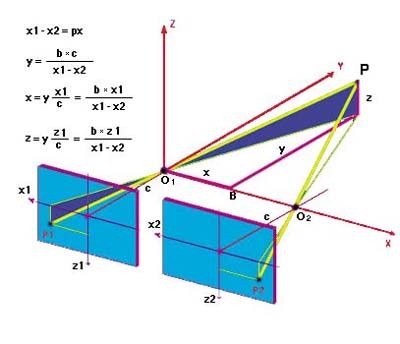The geometric scheme of the "normal case", represented in three-dimensional space (x, y, z)
Always using the similarities of the triangles, it is possible to obtain three formulas, shown in the figure, which establish a one-to-one correspondence between the photo-coordinates and the object-coordinates.
In this representation it is assumed that the photographic recording is carried out with a single camera and, therefore, that the "principal distance C" is the same on the right and on the left. In the case of different “principal distances C” the formulas will be different.